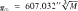# Parallax, Solar

Also found in: Dictionary, Thesaurus.
Related to Parallax, Solar: solar cell
The following article is from The Great Soviet Encyclopedia (1979). It might be outdated or ideologically biased.

## Parallax, Solar

(the sun’s mean equatorial horizontal parallax), the angle subtended by the equatorial radius of the earth at the mean distance of the sun. Until radar methods for determining distances to the planets were introduced into astronomy, the numerical value of the solar parallax was one of the most important fundamental astronomical constants, since, in combination with the geodetically measured equatorial radius of the earth, it was used to determine the value in kilometers of the astronomical unit (AU), which served as a basic unit of linear measure for the universe. There are three types of methods for determining the solar parallax: geometric (trigonometric), dynamical (gravitational), and physical.

Geometric methods. The geometric methods for determining the solar parallax are based on precise astrometrical measurements of the positions of the planets relative to the stars. From two observatories that lie on almost the same meridian and that are at sufficiently different latitudes, the declination of a particular planet is determined by means of meridian or vertical circles; in this way the equatorial horizontal parallax of the planet is computed. Knowing the periods of revolution of the observed planet and the earth, we can compute the solar parallax on the basis of Kepler’s third law. The parallax of a planet can also be determined at a single observatory by measuring the planet’s position relative to the stars with a heliometer at different times of day, thus taking advantage of the observer’s movement in space owing to the earth’s diurnal rotation. Observations of Mars were made for this purpose from the second half of the 17th century, since at favorable oppositions Mars is 0.37 AU from the earth. At such a close opposition, the parallax of Mars is 2.5 times larger than that of the sun. Even more precise determinations of the solar parallax are afforded by meridian and heliomet-ric observations of asteroids, whose positions on the celestial sphere can be computed more reliably owing to their starlike image. Photographic observations of asteroids that come quite close to the earth have been used for the determination of the solar parallax since the end of the 19th century. Such asteroids include Eros, which at its closest approach is 1/7 AU from the earth with a parallax equal to 60”, Icarus, and Geographos. Following the ideas of J. Kepler, astronomers in the 18th and 19th centuries, observed the transit of Venus across the sun’s disk in order to determine the solar parallax. They measured at two observatories the time Venus takes to cross the solar disk. The theory behind this method had been worked out in 1677 by E. Halley.

Dynamical methods. The dynamical methods for determining the solar parallax are based on the study of perturbations in the motion of the moon and planets caused by the attraction of other celestial bodies. The relation between the solar parallax π/⊙ and the combined mass M of the earth and moon, expressed in solar mass units, is given by the equationwhich follows from Kepler’s third law. If the total mass of the earth and moon is known, the solar parallax can be calculated from the perturbations these bodies cause in the motion of a planet. Other dynamical methods for determining the solar parallax exist as well.

Physical methods. The physical methods of determining the solar parallax are based, for example, on the relation between the earth’s mean orbital velocity Vo, which is approximately 29.8 km/sec, and the semimajor axis a of the earth’s orbit (a is approximately equal to 1 AU). The velocity V0 can be determined by measuring the radial velocity of stars lying near the ecliptic; by determining the annual constant of aberration κ, which is equal to the ratio of V0 to the velocity of light; or by measuring the Doppler shift of the lines with wavelength of 21 cm in the spectra of interstellar hydrogen clouds. The radar methods that have been developed for measuring distances from the earth to the moon, planets, and space probes, as well as the Doppler frequency-shift method, have made possible direct determinations of the value of the astronomical unit in kilometers. In the system of astronomical constants that was adopted in 1964, the astronomical unit is equal to 149.6 million km. Thus, in this system the solar parallax is a derived astronomical constant and equals 8.794”. The light time for 1 AU, that is, the time in which light travels a distance of 1 AU, is taken as τA = 499.012 sec, and the equatorial radius of the earth’s spheroid as 6378.160 km.

### REFERENCES

Blazhko, S. N. Kurs sfericheskoi astronomii, 2nd ed. Moscow-Leningrad, 1954.
Idel’son, N. I. “Fundamental’nye postoiannye astronomii i geodezii.” In Astronomicheskii ezhegodnik SSSR na 1942 god. Moscow-Leningrad, 1941, pp. 431–34.
Kulikov, K. A. Fundamental’nye postoiannye astronomii. Moscow, 1956.
Fundamental’nye postoiannye astronomii. [Proceedings of the 21st Symposium of the International Astronomical Union, Aug. 21, 1961.] Moscow, 1967.
Lilley, E., and D. Brouwer. “The Solar Parallax and the Hydrogen Line.” The Astronomical Journal, 1959, vol. 64, no. 8.

V. K. ABALAKIN